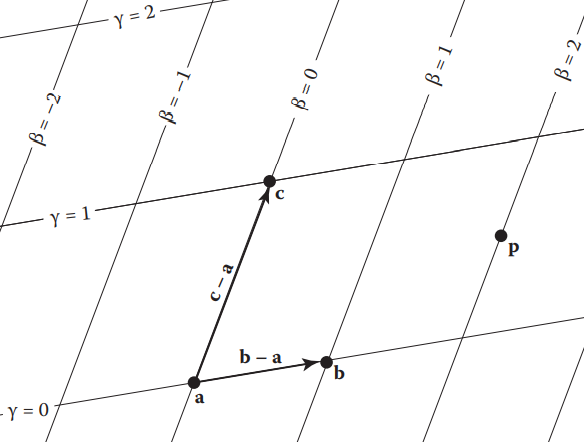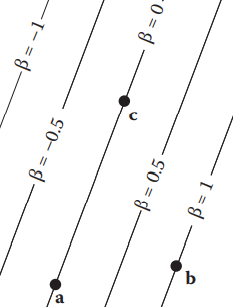# 隐式曲线曲面与梯度、三角形与重心坐标

## 隐式曲线曲面

2D曲线最符合直觉的定义可以是：在一张纸上一笔所画出的一条线，而这条线可以表示为一系列点的集合。对于一般情形，用一个方程可以描述任意二维实曲线：

$f(x,y)=0$

$Ax+By+C=0$

$Ax^2+Bxy+Cy^2+Dx+Ey+F=0$

$f(\textbf{p})={(x-x_c)^2\over a^2}+{(y-y_c)^2\over b^2}-1=0$

3D隐式曲面与2D隐式曲线类似，可用隐函数

$f(x,y,z)=0$

$f(\textbf{p})=0$

$(p-a)\cdot\textbf{n}=0$

3D隐式二次曲面如椭球面：

$f(\textbf{p})={(x-x_c)^2\over a^2}+{(y-y_c)^2\over b^2}+{(z-z_c)^2\over c^2}-1=0$

\left\{ \begin{aligned} f(\textbf{p})&=0\\ g(\textbf{p})&=0 \end{aligned} \right.

## 梯度

${\partial f\over\partial x}=\lim_{\Delta x\rightarrow0}{f(x+\Delta x,y)-f(x,y)\over\Delta x}$

$$l$$​ 是 $$xOy$$ 平面上以 $$P_0(x_0,y_0)$$ 为始点的一条射线$$e_l=(\cos\alpha,\cos\beta)$$ 是与 $$l$$ 同方向的单位向量 (方向余弦表示)，射线 $$l$$​ 的参数方程为

\left\{ \begin{aligned} x&=x_0+t\cos\alpha\\ y&=y_0+t\cos\beta \end{aligned} \right.

$\left.{\partial f\over\partial l}\right|_{(x_0,y_0)}=\lim_{t\rightarrow0^+}{f(x_0+t\cos\alpha,y_0+t\cos\beta)-f(x_0,y_0)\over t}$

\begin{aligned} &f(x_0+\Delta x,y_0+\Delta y)-f(x_0,y_0)\\ =&f_x(x_0,y_0)\Delta x+f_y(x_0,y_0)\Delta y+o\left(\sqrt{(\Delta x)^2+(\Delta y)^2} \right)\\ =&f_x(x_0,y_0)\cos\alpha+f_y(x_0,y_0)\cos\beta+o\left(t\right) \end{aligned}

$\left.{\partial f\over\partial l}\right|_{(x_0,y_0)}=f_x(x_0,y_0)\cos\alpha+f_y(x_0,y_0)\cos\beta$

$\mathrm{grad}f(x_0,y_0)=\nabla f(x_0,y_0)=f_x'\ \textbf{i}+f_y'\ \textbf{j}$

$\left.{\partial f\over\partial l}\right|_{(x_0,y_0)}=(f_x',f_y')\cdot e_l=\left|\mathrm{grad}f(x_0,y_0)\right|\cos\theta$

\left\{ \begin{aligned} z&=f(x,y)\\ z&=c \end{aligned} \right.

\left\{ \begin{aligned} x&=x\\ y&=y(x) \end{aligned} \right.

$f_x(x,y)=f_x(x,y(x))=f_x'+f_y'\ y_x'=(f_x',f_y')\cdot(1,y_x')=0$

$\nabla f(x,y)=({\partial f\over\partial x},{\partial f\over\partial y})$

$\textbf{n}=\nabla f(\textbf{p})=\left({\partial f\over\partial x},{\partial f\over\partial y},{\partial f\over\partial z} \right)$

## 参数曲线曲面

2D参数曲线通常定义为

\left\{ \begin{aligned} x&=g(t) \\ y&=h(t) \end{aligned} \right.

\left\{ \begin{aligned} x&=R\cos\theta\\ y&=R\sin\theta \end{aligned} \right.

3D参数曲线通常定义为

\left\{ \begin{aligned} x&=f(t)\\ y&=g(t)\\ z&=h(t) \end{aligned} \right.

\left\{ \begin{aligned} x&=\cos t\\ y&=\sin t\\ z&= t \end{aligned} \right.

3D参数曲面通常定义为

\left\{ \begin{aligned} x&=f(u,v)\\ y&=g(u,v)\\ z&=h(u,v) \end{aligned} \right.

\left\{ \begin{aligned} x&=R\cos\phi\sin\theta\\ y&=R\sin\phi\sin\theta\\ z&=R\cos\theta \end{aligned} \right.

## 三角形与重心坐标$\textbf{p}=\textbf{a}+\beta(\textbf{b}-\textbf{a})+\gamma(\textbf{c}-\textbf{a})$

$$\alpha=1-\beta-\gamma$$ ，整理得一个对称表达的形式

$\textbf{p}=\alpha\textbf{a}+\beta\textbf{b}+\gamma\textbf{c}$

$$(\alpha,\beta,\gamma)$$ 称为点 $$\textbf{p}$$ 的重心坐标。

• 若坐标分量均位于 $$(0,1)$$ ，则点在三角形内
• 若坐标分量恰有一个为0，且其余两个均位于 $$(0,1)$$ ，则点在三角形上
• 若坐标分量有两个为0，则点与三角形顶点重合
• 除此之外，点在三角形外$\beta={f_{ac}(x,y)\over f_{ac}(x_b,y_b)}$

$\beta={(y_a-y_c)x+(x_c-x_a)y+x_ay_c-x_cy_a\over(y_a-y_c)x_b+(x_c-x_a)y_b+x_ay_c-x_cy_a}$

$\beta={A_b\over A}$

$\textbf{n}=(b-a)\times(c-a)\\ A={1\over2}||\textbf{n}||$

$\textbf{n}_a=(c-b)\times(p-b)$

$\alpha={A_{signed,a}\over A_{signed}}={{1\over2}||\textbf{n}_{a}||\cdot signed(\textbf{n}_a)\over{1\over2}||\textbf{n}||\cdot signed(\textbf{n})}={||\textbf{n}||\ ||\textbf{n}_{a}||\cdot \left<\textbf{n},\textbf{n}_a\right>\over||\textbf{n}||\ ||\textbf{n}||\cdot \left<\textbf{n},\textbf{n}\right>} ={\textbf{n}\cdot\textbf{n}_a\over\textbf{n}\cdot\textbf{n}}$

## 参考

Fundamental of Computer Graphics 4th Edition, Chapter 2.

posted @ 2021-08-25 23:44  Vel'Koz  阅读(417)  评论(0编辑  收藏  举报